# Integral transform

Jump to: navigation, search

A transform of functions, having the form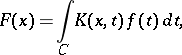(1)

whereis a finite or infinite contour in the complex plane and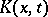is the kernel of the integral transform (cf. Kernel of an integral operator). In most cases one considers integral transforms for which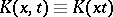andis the real axis or a part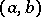of it. If, then the transform is said to be finite. Formulas enabling one to recover the functionfrom a knownare called inversion formulas of the integral transform.

Examples of integral transforms. The Bochner transform: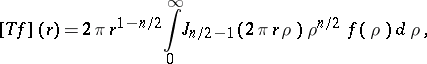whereis the Bessel function of the first kind of order(cf. Bessel functions) andis the distance in. The inversion formula is:. The Parseval identity is:The Weber transform: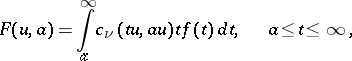where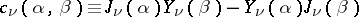and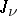and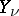are the Bessel functions of first and second kind. The inversion formula is: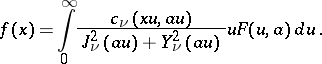For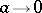, the Weber transform turns into the Hankel transform: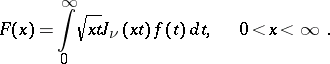For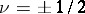this transform reduces to the Fourier sine and cosine transforms. The inversion formula is as follows: If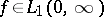, ifis of bounded variation in a neighbourhood of a point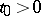and if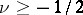, then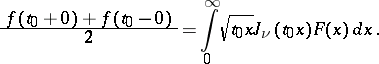The Parseval identity: If, ifandare the Hankel transforms of the functionsand, where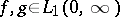, then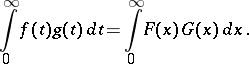Other forms of the Hankel transform are:The Weierstrass transform:it is a special case of a convolution transform.

Repeated transforms. Let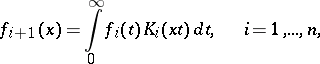where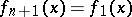. Such a sequence of integral transforms is called a chain of integral transforms. For, repeated integral transforms are often called Fourier transforms.

Multiple (multi-dimensional) integral transforms are transforms (1) where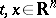andis some domain in the complex Euclidean-dimensional space.

Integral transforms of generalized functions can be constructed by the following basic methods:

1) One constructs a space of test functionscontaining the kernelof the integral transformunder consideration. Then the transform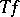for any generalized function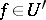is defined as the value ofon the test functionaccording to the formula2) A space of test functionsis constructed on which the classical integral transformis defined, mappingonto some space of test functions. Then the integral transform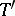of a generalized function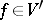is defined by the equation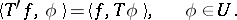3) The required integral transform is expressed in terms of another integral transform that is defined for generalized functions.